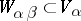# Algebraic variety

(diff) ← Older revision | Latest revision (diff) | Newer revision → (diff)

One of the principal objects of study in algebraic geometry. The modern definition of an algebraic variety as a reduced scheme of finite type over a fieldis the result of a long evolution. The classical definition of an algebraic variety was limited to affine and projective algebraic sets over the fields of real or complex numbers (cf. Affine algebraic set; Projective algebraic set). As a result of the studies initiated in the late 1920s by B.L. van der Waerden, E. Noether and others, the concept of an algebraic variety was subjected to significant algebraization, which made it possible to consider algebraic varieties over arbitrary fields. A. Weil  applied the idea of the construction of differentiable manifolds by glueing to algebraic varieties. An abstract algebraic variety is obtained in this way and is defined as a systemof affine algebraic sets over a field, in each one of which open subsets, corresponding to the isomorphic open subsets, are chosen. All basic concepts of classical algebraic geometry could be transferred to such varieties. Examples of abstract algebraic varieties, non-isomorphic to algebraic subsets of a projective space, were subsequently constructed by M. Nagata and H. Hironaka , . They used complete algebraic varieties (cf. Complete algebraic variety) as the analogues of projective algebraic sets.

J.-P. Serre  has noted that the unified definition of differentiable manifolds and analytic spaces as ringed topological spaces has its analogue in algebraic geometry as well. Accordingly, algebraic varieties were defined as ringed spaces (cf. Ringed space), locally isomorphic to an affine algebraic set over a fieldwith the Zariski topology and with a sheaf of germs of regular functions on it. The supplementary structure of a ringed space on an algebraic variety makes it possible to simplify various constructions with abstract algebraic varieties, and study them using methods of homological algebra which involve sheaf theory.

At the International Mathematical Congress in Edinburgh in 1958, A. Grothendieck outlined the possibilities of a further generalization of the concept of an algebraic variety by relating it to the theory of schemes. After the foundations of this theory had been established  a new meaning was imparted to algebraic varieties — viz. that of reduced schemes of finite type over a field, such affine (or projective) schemes became known as affine (or projective) varieties (cf. Scheme; Reduced scheme). The inclusion of algebraic varieties in the broader framework of schemes also proved useful in a number of problems in algebraic geometry (resolution of singularities; the moduli problem, etc.).

Another generalization of the concept of an algebraic variety is related to the concept of an algebraic space.

Any algebraic variety over the field of complex numbers has the structure of a complex analytic space, which makes it possible to use topological and transcendental methods in its study (cf. Kähler manifold).

Many problems in number theory (the theory of congruences, Diophantine equations, modular forms, etc.) involve the study of algebraic varieties over finite fields and over algebraic number fields (cf. Algebraic varieties, arithmetic of; Diophantine geometry; Zeta-function in algebraic geometry).

How to Cite This Entry:
Algebraic variety. Encyclopedia of Mathematics. URL: http://encyclopediaofmath.org/index.php?title=Algebraic_variety&oldid=14145
This article was adapted from an original article by I.V. Dolgachev (originator), which appeared in Encyclopedia of Mathematics - ISBN 1402006098. See original article